# US20030193617A1 - Equalizer system including a comb filter in an HDTV system - Google Patents

Equalizer system including a comb filter in an HDTV system Download PDF

## Info

Publication number
US20030193617A1
US20030193617A1 US10/388,679 US38867903A US2003193617A1 US 20030193617 A1 US20030193617 A1 US 20030193617A1 US 38867903 A US38867903 A US 38867903A US 2003193617 A1 US2003193617 A1 US 2003193617A1
Authority
US
United States
Prior art keywords
signal
filter
symbols
amplitude
means
Prior art date
Legal status (The legal status is an assumption and is not a legal conclusion. Google has not performed a legal analysis and makes no representation as to the accuracy of the status listed.)
Granted
Application number
US10/388,679
Other versions
US7092036B2 (en
Inventor
Original Assignee
Thomson Licensing SAS
Priority date (The priority date is an assumption and is not a legal conclusion. Google has not performed a legal analysis and makes no representation as to the accuracy of the date listed.)
Filing date
Publication date
Priority to US37291402P priority Critical
Application filed by Thomson Licensing SAS filed Critical Thomson Licensing SAS
Assigned to THOMSON LICENSING S.A. reassignment THOMSON LICENSING S.A. ASSIGNMENT OF ASSIGNORS INTEREST (SEE DOCUMENT FOR DETAILS). Assignors: DSOUZA, ADOLF
Priority to US10/388,679 priority patent/US7092036B2/en
Publication of US20030193617A1 publication Critical patent/US20030193617A1/en
Assigned to THOMSON LICENSING reassignment THOMSON LICENSING ASSIGNMENT OF ASSIGNORS INTEREST (SEE DOCUMENT FOR DETAILS). Assignors: THOMSON LICENSING S.A.
Publication of US7092036B2 publication Critical patent/US7092036B2/en
Application granted granted Critical
Expired - Fee Related legal-status Critical Current

• 238000001914 filtration Methods 0 claims description 11
• 230000003044 adaptive Effects 0 claims description 9
• 238000000034 methods Methods 0 claims description 8
• 238000005311 autocorrelation function Methods 0 claims description 5
• 230000002452 interceptive Effects 0 claims 12
• 230000000875 corresponding Effects 0 claims 2
• 230000002238 attenuated Effects 0 claims 1
• 230000003247 decreasing Effects 0 description 5
• 230000000694 effects Effects 0 description 4
• 238000004364 calculation methods Methods 0 description 2
• 238000004088 simulation Methods 0 description 2

## Images

•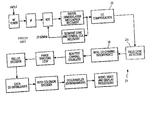•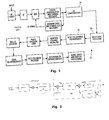•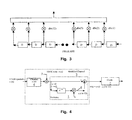•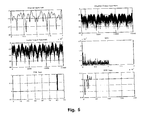•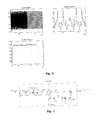•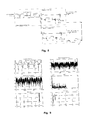•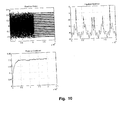•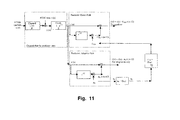•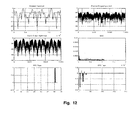•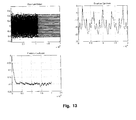## Classifications

• HELECTRICITY
• H04ELECTRIC COMMUNICATION TECHNIQUE
• H04NPICTORIAL COMMUNICATION, e.g. TELEVISION
• H04N5/00Details of television systems
• H04N5/14Picture signal circuitry for video frequency region
• H04N5/21Circuitry for suppressing or minimising disturbance, e.g. moiré or halo
• H04N5/211Ghost signal cancellation

## Abstract

An equalizer (34) and a prefilter (1) used in conjunction with a comb filter (2) in a High Definition Television (HDTV) system. The prefilter (1) may be placed before the comb filter (2), or alternatively may be placed after the comb filter. Further, the method may be enhanced by estimating the filter tap when the prefilter (1) is placed after the comb filter (2). The equalizer (34) produces a fifteen level output from the eight level Vestigial Sideband (VSB) input without limiting the amplitude at symbol 12 to a relatively small value, such as −20 dB. Three specific methods of increasing the permissible amplitude at symbol 12 are presented which permit tap 12 amplitudes as high as −6 dB.

## Description

• The present patent application claims priority from provisional patent application No. 60/372,914 filed on Apr. 16, 2002.
• BACKGROUND OF THE INVENTION
• 1. Field of the Invention 
• This invention relates generally to the field of digital signal processing and more particularly to an equalizing and filtering system suitable for processing eight symbol vestigial sideband (VSB) High Definition Television (HDTV) signals. 
• 2. Background of the Invention 
• The Advanced Television Systems Committee (ATSC) standard for HDTV in the United States specifies an eight bit (eight levels per symbol) vestigial sideband (VSB) transmission system. A block diagram of a typical ATSC compatible HDTV receiver  21 is depicted in FIG. 1. The specifications for such an HDTV receiver can be found in the publication Digital Television Standards for HDTV Transmission of Apr. 12, 1995 prepared by the ATSC. The received VSB signal is represented by a one dimensional data symbol constellation in which only one axis contains quantized data to be recovered by the receiver. A DC compensation unit 26 uses an adaptive tracking circuit to remove from the demodulated VSB signal a DC offset component to the pilot signal component. Field sync detector 28 recovers the data field sync component by comparing every received data segment with an ideal field reference signal stored in memory in the receiver. In addition to field synchronization, the field sync signal provides a training signal for the channel equalizer 34.
• In the ATSC VSB receiving system, NTSC interference detection and rejection are performed by unit  16. When strong co-channel NTSC interference is detected by unit 16, a comb filter is activated to process the signal prior to reaching the adaptive channel equalizer 34. The adaptive equalizer 34 equalizes both channel amplitude and phase distortion. As depicted in FIG. 2, the equalizer structure that is most commonly utilized includes a Feed Forward Equalizer (FFE) that is used in conjunction with a Decision Feedback Equalizer (DFE). The FFE section is used to equalize preghost signals and channel zeroes that reside outside of the unit circle of the data symbol constellation. The DFE section depicted in FIG. 3 is used to equalize post ghost signals.
• A method that is commonly used to train the equalizer  34 is to apply the Blind Equalization technique until the equalizer has reached some predetermined degree of convergence, at which time the training method is switched to the Decision Directed Equalization technique. The most common form of Blind Equalization is the well known Constant Modulus Algorithm (CMA). Using the CMA, the FFE and DFE coefficients are updated using the following equations: Cn+1 = Cn + αF * e(n) * XFn (FFE update) Dn+1 = Dn + αD * e(n) * XDn (DFE update)
• where: 
• C n=FFE coefficient vector
• D n=DFE coefficient vector
• X Fn=Data vector into the FFE
• X Dn=Data vector into the DFE
• e(n)=Blind equalization error. 
• The blind equalization error is calculated according to the equation: 
• e(n)=y 0(n)*(|y 0(n)|2 −R 2)
• where: 
• y 0(n)=Equalizer output (FFE+DFE output)
• R 2=Ring value squared
• =E(s 4(n)/E(s2(n))
• s(n)=eight VSB constellation points. 
• The comb filter  2 has a transfer function of Hc(z)=1−z−12. The comb filter attenuates the picture and sound carriers of the adjacent NTSC channel. The new signal that the equalizer 34 must then process is the original channel convolved with the comb filter. When the comb filter is inserted prior to the equalizer 34 and the DFE taps are adapted using the CMA, the equalizer 34 produces an eight level output. In effect, the equalizer 34 equalizes both the channel and the comb filter signals. However, the goal is cause the equalizer 34 to produce a fifteen level output by equalizing only the channel signal and not equalizing the comb filter signal.
• U.S. patent application Ser. No. 10/103,055, filed on Apr. 16, 2002, describes a method in which the equalizer  34 produces a fifteen symbol output when a comb filter is inserted to reject the NTSC interference. The described method solves the problem of obtaining a fifteen level output by zeroing tap twelve, dfe(12) of the Decision Feedback Equalizer 34. The limitation of this method is that the channel amplitude at symbol twelve, c12, must be small, typically on the order of less than −20 dB.
• BRIEF SUMMARY OF THE INVENTION
• The present invention addresses the problem associated with prior methods of producing a fifteen level output from an equalizer preceded by a comb filter in an HDTV system. The present invention utilizes a prediction filter to permit amplitudes as high as −6 dB at tap twelve of the Decision Feedback Equalizer (DFE). One embodiment of the present invention modifies the channel impulse response at tap twelve (symbol twelve) of the DFE by placing a predictor prefilter before the comb filter. This arrangement causes the comb filter to perceive the combined channel and prefilter as the modified channel. The comb filter attenuates the NTSC interference and the equalizer therefore senses the remaining signal as being free of any NTSC components. This embodiment tends to achieve the goal of making the amplitude of the symbol twelve signal of the modified channel as small as possible, preferably approaching zero, by appropriate selection of the prefilter coefficients. In a second embodiment, the predictor filter is placed after the comb filter. A third embodiment of the present invention places the predictor filter after the comb filter with an estimate of the equalizer tap amplitude value.
• BRIEF DESCRIPTION OF THE DRAWING
• FIG. 1 is a simplified block diagram of a prior art HDTV receiving system; 
• FIG. 2 is a simplified block diagram showing details of the NTSC co-channel interference filter and adaptive channel equalizer depicted in FIG. 1; 
• FIG. 3 is a simplified block diagram of the Decision Feedback Equalizer depicted in FIG. 2; 
• FIG. 4 is a simplified block diagram of a first embodiment of an equalizing and filtering system constructed according to the principles of the present invention; 
• FIG. 5 is a series of convergence curves showing the characteristics of a channel processed by the first embodiment of the present invention; 
• FIG. 6 is a series of convergence curves depicting the behavior of the equalizer for the case presented in FIG. 5; 
• FIG. 7 is a simplified block diagram of a prior art arrangement in which a predictor filter is placed after a comb filter; 
• FIG. 8 is a simplified block diagram of a second embodiment of the present invention; 
• FIG. 9 is a series of convergence curves showing the characteristics of a channel processed by the second embodiment of the present invention; 
• FIG. 10 is a series of convergence curves depicting the behavior of the equalizer for the case presented in FIG. 9; 
• FIG. 11 is a simplified block diagram of a third embodiment of the present invention; 
• FIG. 12 is a series of convergence curves showing the characteristics of a channel processed by the third embodiment of the present invention; and 
• FIG. 13 is a series of convergence curves depicting the behavior of the equalizer for the case presented in FIG. 12.
• DETAILED DESCRIPTION OF THE INVENTION
• Referring to FIG. 4, a simplified block diagram of the present invention shows a first embodiment of the present invention. One effect of setting the amplitude of symbol twelve at zero, that is, dfe(12)=0.0, during the blind equalization process is that the channel impulse response must have a small value at symbol twelve. Since the value at symbol twelve is not in fact always small, the channel impulse response at symbol twelve is modified by placing a prediction prefilter  1 before the comb filter 2. This arrangement causes the comb filter 2 to perceive the modified channel 3 as a composite of the value of channel 4 and the value of the prefilter 1. By appropriate selection of the coefficients of the prefilter 1, symbol twelve of the modified channel 3 can be made to approach a value of zero.
• If the channel  4 has a transfer function of Hc(z) and the prefilter 1 has a transfer function of Hp(z)=(1−az−12), then the modified channel 3 will have a transfer function of Hcm(z)=Hc(z)(1−az−12). For the case where Hc(z)=(1+c12z−12+ . . . ), the modified channel 3 transfer function Hcm(z) becomes: $H cm  ( z ) = ( 1 + c 12  z - 12 +  … ) - az - 12  ( 1 + c 12  z - 12 +  … ) = … + ( c 12 - a )  z - 12 +  …$
• The modified channel  3 now has a residual value of (c12−a) at symbol twelve. The value of “a” is selected to make this residual value as small as possible.
• An estimate of the signal  5, which has a value of y(n), is made by examining the value ay(n−12) of signal 6 and subtracting that value from y(n). The estimated error, e(n) is then defined as:
• e(n)=y(n)−ay(n−12),
• where a=the prediction coefficient of the prediction filter  1.
• The prediction coefficient “a” is selected to minimize the Mean Square Error (MSE) of e(n), where  $MSE = E  [ e 2  ( n ) ] = E  [ y  ( n ) - ay  ( n - 12 ) ] 2 = E  [ y 2  ( n ) + a 2  y 2  ( n - 12 ) - 2  ay  ( n )  y  ( n - 12 ) ] = φ yy  ( 0 ) + a 2  φ yy  ( 0 ) - 2  a   φ yy  ( 12 ) = [ 1 - a 2 ]  φ yy  ( 0 ) - 2  a   φ yy  ( 12 )$
• The MSE is seen to be a quadratic function of “a”. The minimum MSE (MMSE) is obtained by setting the derivative of the MSE to zero and solving for “a”, thereby producing the optimum predictor coefficient. The resulting value is given as 
• a=Φ yy(12)/Φyy(0).
• Since the MSE is a quadratic function of “a”, the value of “a” that produces the MMSE may be obtained in an iterative fashion using a gradient algorithm. In a preferred embodiment, the Least Mean Squares (LMS) algorithm is used to obtain the predictor coefficient. 
• The channel output value 5, including the NTSC component, is given by: 
• y(n)=x(n)*c(n)+t(n)
• where 
• x(n)=8 VSB symbols 
• c(n)=channel 
• t(n)=NTSC tone. 
• The autocorrelation function Φ yy(m) of y(n), is given by: $φ yy  ( m ) =  E  [ y  ( n )  y  ( n - m ) ] =  E [ ( ∑ k  c  ( k )  x  ( n - k ) ) + t  ( n ) ] [ ( ∑ j  c  ( j )  x  ( n - j - m ) ) +  t  ( n - m ) ] =  E  [ ∑ k  ∑ j  c  ( k )  c  ( j )  x  ( n - k )  x  ( n - j - m ) ] +  E  [ t  ( n )  ∑ j  c  ( j )  x  ( n - j - m ) ] + E  [ t  ( n - m )  ∑ k  c  ( k )  x  ( n - k ) ] +  E  [ t  ( n )  t  ( n - m ) ] =  σ x 2  φ cc  ( m ) + 0 + 0 + E  [ t  ( n )  t  ( n - m ) ] ,$
• assuming that the 8 level VSB signal, x(n), is white and that the NTSC tone and the 8 level VSB symbols are uncorrelated, where Φ cc(m) is the autocorrelation function of the channel. If t(n)=A cos (w0n), then $E [ t  ( n )  t  ( n - m ) =  E  [ A 2  cos  ( w 0  n )  cos  ( w 0 )  ( n - m ) ] =  E  [ A 2 / 2  { cos  ( w 0  m ) + cos  ( 2  w 0  n - w 0  m ) } ] =  ( A 2 / 2 )  cos  ( w 0  m ) ,$
• where A=NTSC tone 7 amplitude. 
• The autocorrelation function then becomes: 
• Φyy(m)=σx 2Φcc(m)+(A 2/2)cos(w 0 m).
• For VSB values at the symbol rate of 10.76 MegaHertz (MHz), an NTSC tone frequency of 0.8967 MHz and a value of m=12, the autocorrelation function is: 
• Φyy(12)=σx 2Φcc(12)+0.5A 2.
• By defining β as 0.5A 2, for NTSC tone amplitudes of A=0.10 and 0.15 the value of β is 5×10−3 and 11.25×10−3, respectively. Each of these values is relatively small, and result in a ratio of NTSC to VSB power of approximately −13 dB and −10 dB, respectively.
• Based on the foregoing, the optimum predictor coefficient can be stated as:  $a = φ yy  ( 12 ) / φ yy  ( 0 ) = [ σ x 2  φ cc  ( 12 ) + β ] / [ σ x 2  φ cc  ( 0 ) + β ] = [ φ cc  ( 12 ) / φ cc  ( 0 ) ]  F$
• where F=[1+{β/σ x 2Φcc(12)}]/[1+{β/σx 2Φcc(0)}].
• If no NTSC component is present, then β=0 and F=1, and the optimum predictor coefficient becomes Φ cc(12)/Φcc(0). F is the factor by which the optimum predictor coefficient without an NTSC component is modified due to the presence of the NTSC component 7.
• In order to more fully explain the foregoing, the simulation results for several specific cases will now be presented and examined. Table 1 depicts the assumed ghost signal profiles that pertain to the following discussion.  TABLE 1 Channel Amplitude Channel Delay (symbols) (absolute) A 0 1.0 B 0 1.0 5 0.5 C 0 1.0 5 0.5 20  0.3 D 0 1.0 5 0.5 15  0.3 20  0.3 E 0 1.0 5 0.5 15  0.3 20  0.3 24  0.3
• Table 2 depicts the assumed signal characteristics of channel D.  TABLE 2 Constellation Points −224, −160, −96, −32, 32, 96, 160, 224. (All divided by 512) Ring Size (fifteen level ring) 0.3125 Blind Equalization (CMA) - One Million Number of symbols Decision Directed Equalization One Million (DDE) - Number of symbols Initial Value of FFE Main Tap 1.0 Comb Gain 0.5 FFE step size 0.001 DFE step size 0.008 Prediction Filter before Comb - Step 0.2 × 10−3 Size Channel D NTSC Tone Amplitude Depicted in FIG. 5
• The first example is for the case where the NTSC signal  7 is equal to zero, in which case the comb filter 2 is not needed. The only contribution to Φcc(12) is from the main channel 4 and from a ghost signal at symbol twelve. In other words, there is no other pair of ghosts that are spaced exactly twelve symbols apart. For this situation, the predictor coefficient is: $a =  φ cc  ( 12 ) / φ cc  ( 0 ) =  c 12 / φ cc  ( 0 ) , the   main   tap   of   the   channel   4   being   equal   to   1.$
• Since Φ cc(0)=1+c12 2+(squared terms attributable to other ghost signals), then
• Φcc(0)>1.
• Therefore, |a|=|c 12|, except in the trivial case where the channel is an impulse. In that case, the value of c12=0 and a=0. This means that the predictor “a” can never converge exactly to c12 and will always converge to a value having a magnitude that is less than c12. The modified channel 3 then has a residual value of (c12−a) at symbol twelve. The magnitude of the residual value determines whether or not Blind Equalization will converge to fifteen levels. The residual value (c12−a) at symbol twelve is equal to c12−[c12cc(0)], which is equal to c12[1−1/Φcc(0)]. This residual value is directly proportional to c12, so for large values of c12 the residual value will be high and the Blind Equalization algorithm will not converge. As the value of c12 is reduced, the residual value will decrease and will at some value be small enough for the Blind Equalization algorithm to converge.
• Further, since Φ cc(0) is a measure of the total channel energy, an increase in total channel energy will cause the residual value at symbol twelve to increase. This means that if a channel has several ghost signals, the residual value will be relatively larger. Thus, in order to have the small residual value necessary to achieve convergence, the value of c12 will necessarily need to be relatively smaller. In other words, as the ghost energy increases, the maximum allowable amplitude at symbol twelve decreases, as shown in Table 3 for channels A, B, C and D. This is not the case for channel E because there is more than one contributor to Φcc(12), due to the correlation between c0, c12 and c12, c24. In summary, with no NTSC component and only one contributor to Φcc(12), the predictor “a” will converge to a value that is less than the magnitude of c12. As c12 decreases, the residual value at symbol twelve in the modified channel 3 also decreases, making the convergence to fifteen levels possible at some point. Finally, as the ghost energy increases, the maximum allowable value of c12 decreases. TABLE 3 NTSC Amplitude = 0.0 Maximum Positive Amplitude Maximum Negative Amplitude Ghost Profile (dB) at channel tap = 12 (dB) at channel tap = 12 Delay Amplitude dfetap (12) Predictor dfetap (12) Predictor Channel (symbols) (absolute) zero in Blind Before Comb zero in Blind Before Comb A  0 1.0 12 −18  −6 −18  −7 B  0 1.0  5 0.5 12 −18  −9 −20  −9 C  0 1.0  5 0.5 12 −20 −11 −20 −11 20 0.3 D  0 1.0  5 0.5 12 −21 −12 −20 −12 15 0.3 20 0.3 E  0 1.0  5 0.5 12 −23 −10 −24 −11 15 0.3 20 0.3 24 0.3
• The second example addresses the case of multiple contributions to the value of Φ cc(12) in the absence of an NTSC signal 7. In this case the prediction coefficient is: $a =  φ cc  ( 12 ) / φ cc  ( 0 ) =  [ c 12 + contributions   from   other   terms ] / φ cc  ( 0 ) =  [ c 12 / φ cc  ( 0 ) ] + [ contributions   from   other   terms / φ cc  ( 0 ) ] ,$
• and the residual value becomes 
• c 12[1−1/Φcc(0)]+[contributions from other terms/Φcc(0)].
• In this case, the factor due to contributions from other terms may move the predictor coefficient towards or away from c 12 depending on whether the contributions have the same or opposite sign as c12, as well as the magnitude of the contributions. Therefore, the likelihood of convergence becomes unpredictable. The equalizer 34 may or may not converge depending on how closely the prediction coefficient approximates the value of c12.
• A third example assumes the presence of an NTSC component and that the contribution to Φ cc(12) is from the main channel and c12 only. As discussed earlier, the optimum predictor coefficient is defined as:
• a=[Φ cc(12)/Φcc(0)]F
• where F=[1+{β/σ x 2Φcc(12)}]/[1+{β/σx 2Φcc(0)}].
• Given the presence of an NTSC component and that the contribution to Φ cc(12) is from the main channel and c12 only, the value of Φcc(12)=c12. If the amplitude at channel symbol twelve is positive, then
• σx 2Φcc(12)<σx 2Φcc(0),
• β/σx 2Φcc(12)>β/σx 2Φcc(0),
• 1+β/σx 2Φcc(12)<1+β/σx 2Φcc(0),
• or, stated differently, F<1. 
• Therefore, with a negative amplitude at channel symbol twelve, c 12, the effect of the NTSC component 7 is to increase the value of the prediction coefficient “a”, keeping in mind that the value of the prediction coefficient is negative because c12 is negative. In other words, the magnitude of the prediction coefficient decreases since it is becoming more positive. Since the prediction coefficient is decreased in magnitude, the value of the prediction coefficient moves farther away from the value of c12 and the residual value at symbol twelve is increased. Similarly, with the NTSC component present, the maximum allowable value at symbol twelve decreases relative to the maximum permissible value in the absence of the NTSC tone.
• In summary, the presence of the NTSC tone  7 affects the value of the predictor coefficient “a”. When C12 is positive, the NTSC component 7 increases the allowable range of c12, while the absence of the NTSC component 7 decreases allowable range of c12. When the NTSC tone 7 is absent, an increase in the allowable magnitude of a positive c12 and a decrease in the allowable magnitude of a negative c12 results in an asymmetry in the permissible values of c12.
• A fourth case assumes the presence of an NTSC component  7 and that the contribution to Φcc(12) is from several sources. In this case, the optimum predictor coefficient is defined as
• a=[(c 12+contributions from other terms)/Φcc(0)]F.
• Again, the contribution from other terms will cause an error in the predictor coefficient. Thus, in this case, the likelihood of convergence is unpredictable. 
• While Table 3 illustrates the performance of the first embodiment of the present invention in the absence of the NTSC component, Table 4 depicts the case where the NTSC amplitude is 0.10, and Table 5 addresses the case where the NTSC amplitude is 0.15. NTSC tone amplitudes values of 0.10 and 0.15 result in NTSC to VSB power ratios of approximately −13 dB and −10 dB, respectively. The simulation parameters are shown in Table 2. The value of dfe(12) is set at zero in the Blind Equalization mode and is allowed to adapt in the Decision Directed Mode. The maximum positive and negative value of c 12 presented in the Table 3, Table 4 and Table 5 is determined by decreasing the amplitude of c12 in one decibel steps until convergence is achieved. The values presented are for both positive and negative values of c12 for every channel. The predictor coefficient “a” is updated for every symbol from the start of the Blind Equalization process using the LMS algorithm. Referring to FIG. 4,
• e(n)=y(n)−ay(n−12),
• a(n+1)=a(n)+alphap*e(n)*y(n−12),
• where 
• a(n)=old predictor coefficient, 
• a(n+1)=updated predictor coefficient, 
• alphap=predictor step size, and 
• y(n)=channel output, including the NTSC component. 
• Convergence curves for Channel D with an NTSC amplitude of 0.15 are shown in FIGS. 5 and 6. The complete results are shown in Table 3, Table 4 and Table 5. The tables also show, for comparison purposes, the results of setting dfe(12) to zero in a Blind Equalization mode without the use of any predictor.  TABLE 4 NTSC Amplitude = 0.10 Maximum Positive Amplitude Maximum Negative Amplitude Ghost Profile (dB) at channel tap = 12 (dB) at channel tap = 12 Amplitude dfetap (12) Predictor dfetap (12) Predictor Channel Delay (symbols) (absolute) zero in Blind Before Comb zero in Blind Before Comb A  0 1.0 12 −18  −5 −19  −9 B  0 1.0  5 0.5 12 −19  −8 −20 −12 C  0 1.0  5 0.5 12 −21 −10 −20 −15 20 0.3 D  0 1.0  5 0.5 12 −21 −10 −20 −15 15 0.3 20 0.3 E  0 1.0  5 0.5 12 −23  −8 −22 −17 15 0.3 20 0.3 24 0.3
•  TABLE 5 NTSC Amplitude = 0.15 Maximum Positive Amplitude Maximum Negative Amplitude Ghost Profile (dB) at channel tap = 12 (dB) at channel tap = 12 Amplitude dfetap (12) Predictor dfetap (12) Predictor Channel Delay (symbols) (absolute) zero in Blind Before Comb zero in Blind Before Comb A  0 1.0 12 −18 −5 −18 −23 B  0 1.0  5 0.5 12 −19 −7 −19 −25 C  0 1.0  5 0.5 12 −21 −9 −20 −28 20 0.3 D  0 1.0  5 0.5 12 −20 −9 −20 −27 15 0.3 20 0.3 E  0 1.0  5 0.5 12 −23 −7 −23 −44 15 0.3 20 0.3 24 0.3
• The first embodiment of the present invention just described, in which the predictor  1 is placed before the comb filter 2, fails to produce a fifteen level output in the presence of a preghost+postghost composite signal that supplies energy to dfe(12), even if the value of c12 is zero. Further, when there are more than two contributors to Φcc(12), the convergence becomes unpredictable. This case occurs when there are two ghost signals spaced twelve symbols apart, other than the ghost at symbol twelve. Finally, a fifteen level output will not be produced if the amplitude of c12 is outside of the allowable range for the particular channel.
• An examination of Table 3, Table 4 and Table 5 shows that the presence of an NTSC component causes the allowable range of c 12 to be asymmetric. The allowable range of c12 is larger with the use of a predictor for lower NTSC tone levels (0.0 and 0.10). For an NTSC level of 0.15, the range of allowable positive c12 values is greater than possible without the use of a predictor, but the allowable range of negative c12 values is decreased, due to the effect of the NTSC tone on the predictor coefficient. The plots of the DFE taps shown in FIGS. 5 and 6 show that the length of the modified channel 3 increases by twelve symbols over the original channel 4, and that more ghosts of a smaller amplitude are introduced because of the predictor. Finally, the NTSC tone 7 is seen to be an advantage to the predictor coefficient if the value of c12 is positive, but is a disadvantage if the value is negative. This characteristic points to the need to cause the predictor coefficient to be independent of the NTSC tone, as will now be discussed.
• In the second embodiment of the present invention, the predictor coefficient “a” is made independent of the NTSC signal by placing the predictor  1 after the comb filter 2. The prior art version of such an arrangement is depicted in FIG. 7. In this arrangement, the comb filter 2 attenuates the NTSC tone 7 and the predictor 1 perceives a signal 8 that is free of the NTSC component. The channel that the predictor 1 receives is the original channel 4 convolved with the comb filter 2. The predictor transfer function is $Hcp  ( z ) =  ( 1 + c 12  z - 12 + … )  ( 1 - z - 12 ) =  1 + ( c 12 - 1 )  z - 12 + …$
• The predictor  1 perceives a value of (c12−1) at symbol twelve and will converge to a value that is related to (c12−1), which is not desired. The goal is to perceive the channel as having only c12 at symbol twelve. As discussed for the first embodiment of the present invention, the predictor 1 will converge to
• a Azz(12)/Φzz(0),
• where a A=the value to which the predictor 1 will converge if the predictor 1 is placed before the comb filter 2. However, the goal is to have the comb filter 2 hidden from the predictor 1. Ideally, the predictor 1 will converge to a value of aByy(12)/Φyy(0) with an NTSC signal 7 of zero, where aB is the value to which the predictor 1 will converge if placed before the comb filter 2 and in the absence of any NTSC signal 7. In other words, the predictor 1 should converge to the same value that would occur if the predictor 1 were placed before the comb filter 2 without any NTSC component 7.
• Thus the relationship between the equations a Azz(12)/Φzz(0) and aByy(12)/Φyy(0) needs to be derived, which is done as follows: $φ zz  ( m ) =  E  [ z  ( n )  z  ( n - m ) ] =  E  [ { y  ( n ) - y  ( n - 12 ) }  { y  ( n - m ) - y  ( n - m - 12 ) } ] =  φ yy  ( m ) - φ yy  ( m + 12 ) - φ yy  ( m - 12 ) + φ yy  ( m ) =  2   φ yy  ( m ) - φ yy  ( m + 12 ) - φ yy  ( m - 12 ) .$
• So Φ zz(0)=2Φyy(0)−2Φyy(12) (autocorrelation is an even function),
• and Φ zz(12)=2Φyy(12)−Φyy(24)−Φyy(0).
• Therefore, the value a A, is
• a A=[2Φyy(12)−Φyy(0)−Φyy(24)]/[2Φyy(0)−2Φyy(12)].
• If the value of Φ yy(24) is assumed to be zero, and knowing that aByy(12)/Φyy(0), then $a A = [ 2  φ yy  ( 12 ) - φ yy  ( 0 ) ] / [ 2  φ yy  ( 0 ) - 2  φ yy  ( 12 ) ] = [ 2  a B - 1 ] / [ 2 - 2  a B ] .$
• Solving for a B produces the following relationship between aA and aB:
• a B=[2a A+1]/[2a A+2].
• Referring also to FIG. 8, the second embodiment of the present invention shows that the predictor  1 can be placed after the comb filter 2 and be permitted to adapt to give a value for aA. The value of aB can then be calculated from aA, and the value of aB can be used in the direct path 9 to the equalizer 34. The maximum positive and negative amplitudes for representative channels A, B, C, D and E are set forth in Table 6, Table 7 and Table 8. The values associated with these channels are the same as shown in Table 2, except that the amplitude of the NTSC tone 7 is 0.15 for all three Table 6, Table 7 and Table 8. The value of dfe(12) is set at zero in the Blind Equalization mode and is allowed to adapt in the Decision Directed mode. The maximum positive and negative value of c12 for each channel is found by decreasing the amplitude of c12 by increments of one decibel until convergence is achieved. Both of the predictor coefficients aA and aB are updated at every symbol. The value of aA is updated using the LMS algorithm, and then aB is calculated from the value of aA using the relationship earlier described. Referring to FIG. 8, the update equations used are as follows:
• Initialize a A to −0.5 so that the initial value of aB is zero.
• r(n)=z(n)−a B z(n−12)
• c(n)=z(n)−a A z(n−12)
• a A(n+1)=a A(n)+alphap*c(n)*z(n−12)
• a B(n+1)=[2a A(n+1)+1]/[2a A(n+1)+2].
• The convergence curves for the case where the NTSC signal  7 amplitude is 0.15 are shown in FIGS. 9 and 10. The predictor coefficient plotted in FIGS. 9 and 10 is aB. Results of the previous two methods are also included in the Table 6, Table 7 and Table 8 for comparison purposes. Note that for channel E, when the predictor 1 is placed after comb filter 2 convergence is not achieved because in deriving the relationship between aA and aB the assumption was made that Φyy(24) was equal to zero. This assumption is violated in the data presented since Φyy(24) is not zero because there is a ghost at symbol 24. When the value of NTSC signal 7 is effectively zero, Φyy(24)=Φcc(24). TABLE 6 NTSC Amplitude = 0.0 Maximum Positive Amplitude (dB) at Maximum Negative Amplitude channel tap = 12 (dB) at channel tap = 12 Ghost Profile Predictor Predictor Predictor Predictor Amplitude dfetap (12) Before After dfetap (12) Before After Channel Delay (symbols) (absolute) zero in Blind Comb Comb zero in Blind Comb Comb A  0 1.0 12 −18  −6  −6 −18  −7  −7 B  0 1.0  5 0.5 12 −18  −9  −9 −20  −9  −9 C  0 1.0  5 0.5 12 −20 −11 −11 −20 −11 −11 20 0.3 D  0 1.0  5 0.5 12 −21 −12 −12 −20 −12 −11 15 0.3 20 0.3 E  0 1.0  5 0.5 12 −23 −10 Did −24 −11 Did not not converge converge 15 0.3 20 0.3 24 0.3
•  TABLE 7 NTSC Amplitude = 0.10 Maximum Positive Amplitude (dB) at Maximum Negative Amplitude channel tap = 12 (dB) at channel tap = 12 Ghost Profile Predictor Predictor Predictor Predictor Amplitude dfetap (12) Before After dfetap (12) Before After Channel Delay (symbols) (absolute) zero in Blind Comb Comb zero in Blind Comb Comb A  0 1.0 12 −18  −5  −6 −19  −9  −7 B  0 1.0  5 0.5 12 −19  −8  −9 −20 −12  −9 C  0 1.0  5 0.5 12 −21 −10 −11 −20 −15 −11 20 0.3 D  0 1.0  5 0.5 12 −21 −10 −12 −20 −15 −11 15 0.3 20 0.3 E  0 1.0  5 0.5 12 −23  −8 Did not −22 −17 Did not converge converge 15 0.3 20 0.3 24 0.3
•  TABLE 8 NTSC Amplitude = 0.15 Maximum Positive Amplitude (dB) at Maximum Negative Amplitude channel tap = 12 (dB) at channel tap = 12 Ghost Profile Predictor Predictor Predictor Predictor Amplitude dfetap (12) Before After dfetap (12) Before After Channel Delay (symbols) (absolute) zero in Blind Comb Comb zero in Blind Comb Comb A  0 1.0 12 −18 −5  −6 −18 −23  −7 B  0 1.0  5 0.5 12 −19 −7  −9 −19 −25  −9 C  0 1.0  5 0.5 12 −21 −9 −11 −20 −28 −11 20 0.3 D  0 1.0  5 0.5 12 −20 −9 −12 −20 −27 −11 15 0.3 20 0.3 E  0 1.0  5 0.5 12 −23 −7 Did not −23 −44 Did not converge converge 15 0.3 20 0.3 24 0.3
• As was discussed with regard to the first embodiment of the present invention, the predictor “a” will converge to a value that is less than the magnitude of c 12, resulting in a residual of (a−c12) at symbol twelve in the modified channel 3.
• A third embodiment of the present invention addresses the problem of estimating c 12 from the predictor coefficient “a” in order to make the residual value smaller. In the case of the first embodiment, the predictor was seen to converge to a value given by a=Φcc(12)/Φcc(0). For the case where there is only one contribution to Φcc(12) from the main channel 4 and c12, the predictor is $a = φ cc  ( 12 ) / φ cc  ( 0 ) = c 12 / ( 1 + c 12 2 + E ) ,$
• where E=energy from ghost signals other than the main channel and c 12.
• If the value of E is zero, as occurs in the unusual case where there is a ghost signal only at symbol twelve, the equation for the predictor becomes a quadratic relationship between “a” and c 12. This relationship permits the calculation of an estimated value for c12. When E has a nonzero value, the equation becomes
• ac 12 2 −c 12 +a(1+E)=0.
• Solving for c 12 gives the result
• c 12 =[1−{square root}{square root over (1−4a 2(1+E))}]/(2a)
• If E=0, as is the case for channel A, then c 12 can be solved exactly, once the prediction coefficient is known, and then c12 can be used in the direct path to the equalizer 34. In most cases, the value of E will not be known and the value of c12 cannot be determined. However, by assuming that E is small, the value of c12 can be calculated from the predictor “a”. By using this assumption, the allowable amplitude of c12 can be increased. The resulting configuration is depicted in FIG. 11 in which an additional tap prediction block 10 appears for the calculation of estimated tap value c12e.
• The maximum positive and negative amplitudes for representative channels A, B, C, D and E are set forth in Table 9, Table 10 and Table 11. The values associated with these channels are the same as shown in Table 2. The value of dfe(12) is set at zero in the Blind Equalization mode and is allowed to adapt in the Decision Directed mode. The maximum positive and negative value of c 12 for each channel is found by decreasing the amplitude of c12 by increments of one decibel until convergence is achieved. Both of the predictor coefficients aA and aB, and the tap estimate c12e are updated at every symbol. Referring to FIG. 11, the update equations used are as follows:
• Initialize a A to −0.5 so that the initial value of aB is zero.
• r(n)=z(n)−c 12e z(n−12)
• c(n)=z(n)−a A z(n−12)
• a A(n+1)=a A(n)+alphap*c(n)*z(n−12)
• a B(n+1)=[2a A(n+1)+1]/[2a A(n+1)+2]
• c 12e(n+1)=[1−{square root}{square root over (1−4a B 2(1+E))}]/(2a B(n+1)
• In order to avoid a square root of a negative number, the absolute value of a B must be less than or equal to 0.5. In order to avoid division by zero, the value of aB must not be zero. Whenever the absolute value of aB is greater than 0.5 or is equal to zero, the value of c12e is not updated but is instead frozen at its most recent value. The convergence curves for three cases are shown in FIGS. 12 and 13. Results of the previous three methods are also included in Table 9, Table 10 and Table 11 for comparison purposes. TABLE 9 NTSC Amplitude = 0.0 Maximum Positive Amplitude (dB) at channel tap = 12 Maximum Negative Amplitude (dB) at Predictor channel tap = 12 Ghost Profile dfetap (12) Predictor After Comb dfetap (12) Predictor After Comb Delay Amplitude zero in Before After with tap zero in Before After with tap Channel (symbols) (absolute) Blind Comb Comb estimate Blind Comb Comb estimate A  0 1.0 12 −18  −6  −6  −1 −18  −7  −7  −1 B  0 1.0  5 0.5 12 −18  −9  −9  −8 −20  −9  −9  −9 C  0 1.0  5 0.5 12 −20 −11 −11 −11 −20 −11 −11 −11 20 0.3 D  0 1.0  5 0.5 12 −21 −12 −12 −11 −20 −12 −11 −11 15 0.3 20 0.3 E  0 1.0  5 0.5 12 −23 −10 Did not converge −24 −11 Did not converge 15 0.3 20 0.3 24 0.3
•  TABLE 10 NTSC Amplitude = 0.10 Maximum Positive Amplitude (dB) at channel tap = 12 Maximum Negative Amplitude (dB) at Predictor channel tap = 12 Ghost Profile dfetap (12) Predictor After Comb dfetap (12) Predictor After Comb Delay Amplitude zero in Before After with tap zero in Before After with tap Channel (symbols) (absolute) Blind Comb Comb estimate Blind Comb Comb estimate A  0 1.0 12 −18  −5  −6  −1 −19  −9  −7  −1 B  0 1.0  5 0.5 12 −19  −8  −9  −8 −20 −12  −9  −9 C  0 1.0  5 0.5 12 −21 −10 −11 −11 −20 −15 −11 −10 20 0.3 D  0 1.0  5 0.5 12 −21 −10 −12 −11 −20 −15 −11 −11 15 0.3 20 0.3 E  0 1.0  5 0.5 12 −23 −8 Did not converge −22 −17 Did not converge 15 0.3 20 0.3 24 0.3
•  TABLE 11 NTSC Amplitude = 0.15 Maximum Positive Amplitude (dB) at channel tap = 12 Maximum Negative Amplitude (dB) at Predictor channel tap = 12 Ghost Profile dfetap (12) Predictor After Comb dfetap (12) Predictor After Comb Delay Amplitude zero in Before After with tap zero in Before After with tap Channel (symbols) (absolute) Blind Comb Comb estimate Blind Comb Comb estimate A  0 1.0 12 −18 −5  −6  −1 −18 −23  −7  −1 B  0 1.0  5 0.5 12 −19 −7  −9  −8 −19 −25  −9  −9 C  0 1.0  5 0.5 12 −21 −9 −11 −11 −20 −28 −11 −10 20 0.3 D  0 1.0  5 0.5 12 −20 −9 −12 −12 −20 −27 −11 −11 15 0.3 20 0.3 E  0 1.0  5 0.5 12 −23 −7 Did not converge −24 −44 Did not converge 15 0.3 20 0.3 24 0.3
• Inspection of Table 9, Table 10 and Table 11 reveals that for channel A, where there is a ghost only at symbol 12, the tap estimate can be calculated exactly from the predictor coefficient since E=0. In this case, the residual can be made to be extremely small or, equivalently, c 12 can take on a very large value (−1 dB). In practice the probability of having channel A is very small. For channels B, C, D and E, the maximum allowable positive and negative amplitudes for c12 are in most cases the same as the method presented in the second embodiment, but in a few instances, the maximum allowable amplitude is 1 dB more.
• The use of the predictor  1 after the comb filter 2 along with the use of the tap estimate will fail to produce a fifteen level output at the equalizer 34 in several cases. One case is when a preghost+postghost composite signal is present that supplies energy to dfe(12), even if the value of c12 is zero. Also, when there are more than two contributors to Φcc(12), the convergence becomes unpredictable. This case occurs when there are two ghost signals spaced twelve symbols apart, other than the ghost at symbol twelve. Another failed case occurs when there is a ghost at symbol 24 or there are two ghosts spaced 24 symbols apart. A fifteen level output will not be produced if the amplitude of c12 is outside of the allowable range for the particular channel. Finally, whenever the absolute value of aB is greater than 0.5, the tap estimate c12e is frozen at its previous value, since the argument of the square root becomes negative. Therefore, a problem will occur whenever the absolute value of the predictor coefficient aB has to converge to a value of greater than 0.5.
• Three specific embodiments have been presented for producing at fifteen level output at the equalizer  34. Of those three, the placement of the predictor 1 after the comb filter 2 is typically the best choice. The scope of the invention, however, is defined by the claims and is not limited to the specific embodiments disclosed.

## Claims (20)

1. In a system for processing a video data signal comprising a sequence of symbols possibly including an interfering signal, an apparatus for providing an output signal having a desired characteristic, comprising:
means for detecting (16) the interfering signal;
a first filter (2) responsive to said interfering signal, said first filter tending to attenuate the interfering signal;
means for predicting (1) a characteristic of said video data signal and generating a modified signal in response to said characteristic; and
means (34) responsive to said first filter (2) and said means for predicting (1) a characteristic of said video data for providing an equalized output signal.
2. A system according to claim 1, wherein said means for predicting (1) a characteristic of said video data signal processes particular data symbols prior to processing of said particular data symbols by the first filter (2).
3. A system according to claim 2, wherein the means for predicting (1) a characteristic of said video data signal generates a modified signal that tends to minimize an amplitude associated with at least one symbol.
4. A system according to claim 1 wherein said first filter (2) processes particular symbols prior to processing of said particular symbols by the means for predicting (1) a characteristic of said video data.
5. A system according to claim 4 wherein the means (34) for providing an equalized output signal is an adaptive equalizer comprising a plurality of tap values corresponding to symbols present in the video data signal.
6. A system according to claim 5, further including means for estimating (10) a tap value utilized by the means (34) for providing an equalized output signal.
7. A system according to claim 6, wherein the means (10) for estimating a tap value causes an amplitude of a selected component of the video data signal to be minimized by the means (34) for providing an equalized output signal.
8. A system according to claim 7, wherein the first filter (2) is a comb filter.
9. In a system for processing video data signal comprising groups of encoded data packets containing a plurality of symbols and possibly containing an interfering signal, a method of obtaining a desired signal characteristic comprising the steps of:
detecting the interfering signal;
filtering the interfering signal;
generating a filter output signal in response to said filtering;
predicting a characteristic of said video data signal from said filter output signal;
generating a modified signal in response to said characteristic of said video data signal; and
equalizing the filter output signal and the modified signal so as to obtain the desired signal characteristic.
10. A system according to claim 9, further comprising the step of prefiltering a signal containing the video data signal and the interfering signal.
11. A system according to claim 10, further comprising the step of selecting prefiltering coefficients such that a selected symbol present in the modified signal has an amplitude approaching a value of zero.
12. A system according to claim 11, further comprising the step of adaptively equalizing the modified signal by means of an adaptive equalizer comprising a plurality of taps, each tap being associated with a particular symbol contained in the encoded data packet.
13. A system according to claim 12, further comprising the step of minimizing a selected symbol amplitude at a selected tap.
14. A system according to claim 12, further comprising the step of estimating a tap value to be utilized by the adaptive equalizer.
15. An equalizing and filtering system for processing video data encoded symbols, comprising:
an input signal, the input signal comprising multiple level vestigial sideband (VSB) symbols;
a prediction filter (1), the prediction filter being responsive to the input signal and an interfering signal, the prediction filter being adapted to minimize an amplitude associated with a selected symbol
a comb filter (2), the comb filter being responsive to an output signal generated by the prediction filter (1); and
an equalizer (34), the equalizer (34) producing a signal having relatively more levels than the multiple level VSB symbol input signal.
16. The equalizing and filtering system of claim 15, wherein the equalizer (34) is an adaptive equalizer comprising a plurality of taps, each tap being associated with a particular symbol.
17. The equalizing and filtering system of claim 16, wherein the prediction filter (1) calculates an optimum prediction coefficient selected to minimize a selected symbol amplitude.
18. The equalizing and filtering system of claim 17, wherein the optimum prediction coefficient is calculated according to the equation
a=[Φ cc(12)/Φcc(0)]F,
where a is the optimum prediction coefficient, Φcc(12) represents an autocorrelation function of a channel containing encoded data symbols, Φcc(0) represents total channel energy for a channel containing encoded data symbols, and F is factor attributable to the interfering signal.
19. An equalizing and filtering system for processing video data encoded symbols, comprising:
an input signal source, the input signal comprising multiple level vestigial sideband (VSB) symbols and containing a desired signal and an interfering signal;
a comb filter (2), the comb filter being responsive to the input signal, the comb filter (2) generating an output signal having an attenuated interfering signal;
a prediction filter (1), the prediction filter being responsive to the output signal generated by the comb filter (2), the prediction filter being adapted to minimize an amplitude associated with a selected symbol; and
an equalizer (34), the equalizer (34) producing a signal having relatively more levels than the multiple level VSB symbol input signal.
20. The equalizing and filtering system of claim 19, wherein the means (34) for providing an equalized output signal is an adaptive equalizer comprising a plurality of tap values corresponding to symbols present in the encoded data packets, the equalizing and filtering system further including means for estimating (10) a tap value utilized by the means (34) for providing an equalized output signal.
US10/388,679 2002-04-16 2003-03-14 Equalizer system including a comb filter in an HDTV system Expired - Fee Related US7092036B2 (en)

## Priority Applications (2)

Application Number Priority Date Filing Date Title
US37291402P true 2002-04-16 2002-04-16
US10/388,679 US7092036B2 (en) 2002-04-16 2003-03-14 Equalizer system including a comb filter in an HDTV system

## Applications Claiming Priority (6)

Application Number Priority Date Filing Date Title
US10/388,679 US7092036B2 (en) 2002-04-16 2003-03-14 Equalizer system including a comb filter in an HDTV system
MXPA03003249 MXPA03003249A (en) 2002-04-16 2003-04-11 Equalizer system including a comb filter in an hdtv system.
MYPI20031386 MY134400A (en) 2002-04-16 2003-04-14 Equalizer system including a comb filter in an hdtv system
JP2003108529A JP4373118B2 (en) 2002-04-16 2003-04-14 Equalizer system and method with comb filter in HDTV system
KR20030023783A KR100982898B1 (en) 2002-04-16 2003-04-15 An equalizer system including a comb filter in an HDTV system
CNB031524559A CN100367790C (en) 2002-04-16 2003-04-15 Equalizing system contg comb filter in HDTV system

## Publications (2)

Publication Number Publication Date
US20030193617A1 true US20030193617A1 (en) 2003-10-16
US7092036B2 US7092036B2 (en) 2006-08-15

# Family

## Family Applications (1)

Application Number Title Priority Date Filing Date
US10/388,679 Expired - Fee Related US7092036B2 (en) 2002-04-16 2003-03-14 Equalizer system including a comb filter in an HDTV system

## Country Status (6)

US (1) US7092036B2 (en)
JP (1) JP4373118B2 (en)
KR (1) KR100982898B1 (en)
CN (1) CN100367790C (en)
MX (1) MXPA03003249A (en)
MY (1) MY134400A (en)

## Cited By (2)

* Cited by examiner, † Cited by third party
Publication number Priority date Publication date Assignee Title
US7248849B1 (en) * 2003-06-03 2007-07-24 Texas Instruments Incorporated Frequency domain training of prefilters for receivers
US20130142520A1 (en) * 2008-06-30 2013-06-06 Chuan Xie Anti-causal pre-emphasis for high speed optical transmission

## Families Citing this family (2)

* Cited by examiner, † Cited by third party
Publication number Priority date Publication date Assignee Title
US7801248B2 (en) * 2004-11-19 2010-09-21 Qualcomm Incorporated Interference suppression with virtual antennas
US7463679B2 (en) * 2005-06-27 2008-12-09 Intel Corporation Equalizer mode selection based on distribution of symbol error

## Citations (7)

* Cited by examiner, † Cited by third party
Publication number Priority date Publication date Assignee Title
US5331416A (en) * 1992-12-02 1994-07-19 Samsung Electronics Co., Ltd. Methods for operating ghost-cancelation circuitry for TV receiver or video recorder
US5512957A (en) * 1994-02-10 1996-04-30 Philips Electronics North America Corp. Method and apparatus for combating co-channel NTSC interference for digital TV transmission
US5602602A (en) * 1994-02-10 1997-02-11 Philips Electronics North America Corporation Method and apparatus for combating co-channel NTSC interference for digital TV transmission having a simplified rejection filter
US5777692A (en) * 1994-12-29 1998-07-07 Philips Electronics North America Corporation Receiver based methods and devices for combating co-channel NTSC interference in digital transmission
US6226049B1 (en) * 1997-03-25 2001-05-01 Samsung Electronics Co., Ltd. NTSC rejection filter
US6384858B1 (en) * 1998-08-24 2002-05-07 Samsung Electronics Co., Ltd. Suppression of co-channel NTSC interference artifacts when extracting training signal for a DTV receiver equalizer
US6826242B2 (en) * 2001-01-16 2004-11-30 Broadcom Corporation Method for whitening colored noise in a communication system

## Family Cites Families (6)

* Cited by examiner, † Cited by third party
Publication number Priority date Publication date Assignee Title
KR960012019B1 (en) * 1993-11-18 1996-09-09 구자홍 Hdtv channel equipment
US6084642A (en) * 1996-10-07 2000-07-04 Zenith Electronics Corporation Receiver for adjusting sync levels in VSB systems
US6011814A (en) 1997-09-30 2000-01-04 Paradyne Corporation Adaptive comb filter and decision feedback equalizer for noise suppression
US6222592B1 (en) * 1998-01-13 2001-04-24 Samsung Electronics Co., Ltd. TV receiver equalizer storing channel characterizations for each TV channel between times of reception therefrom
US6118495A (en) * 1998-01-13 2000-09-12 Samsung Electronics Co., Ltd. Training signal in plural PN sequences near beginnings of data segments of DTV signal or scan lines of NTSC signal
KR100674423B1 (en) * 2001-01-19 2007-01-29 엘지전자 주식회사 Transmitting/receiving system and data processing method

## Patent Citations (7)

* Cited by examiner, † Cited by third party
Publication number Priority date Publication date Assignee Title
US5331416A (en) * 1992-12-02 1994-07-19 Samsung Electronics Co., Ltd. Methods for operating ghost-cancelation circuitry for TV receiver or video recorder
US5512957A (en) * 1994-02-10 1996-04-30 Philips Electronics North America Corp. Method and apparatus for combating co-channel NTSC interference for digital TV transmission
US5602602A (en) * 1994-02-10 1997-02-11 Philips Electronics North America Corporation Method and apparatus for combating co-channel NTSC interference for digital TV transmission having a simplified rejection filter
US5777692A (en) * 1994-12-29 1998-07-07 Philips Electronics North America Corporation Receiver based methods and devices for combating co-channel NTSC interference in digital transmission
US6226049B1 (en) * 1997-03-25 2001-05-01 Samsung Electronics Co., Ltd. NTSC rejection filter
US6384858B1 (en) * 1998-08-24 2002-05-07 Samsung Electronics Co., Ltd. Suppression of co-channel NTSC interference artifacts when extracting training signal for a DTV receiver equalizer
US6826242B2 (en) * 2001-01-16 2004-11-30 Broadcom Corporation Method for whitening colored noise in a communication system

## Cited By (2)

* Cited by examiner, † Cited by third party
Publication number Priority date Publication date Assignee Title
US7248849B1 (en) * 2003-06-03 2007-07-24 Texas Instruments Incorporated Frequency domain training of prefilters for receivers
US20130142520A1 (en) * 2008-06-30 2013-06-06 Chuan Xie Anti-causal pre-emphasis for high speed optical transmission

## Also Published As

Publication number Publication date
JP4373118B2 (en) 2009-11-25
KR100982898B1 (en) 2010-09-20
US7092036B2 (en) 2006-08-15
CN100367790C (en) 2008-02-06
JP2004007577A (en) 2004-01-08
MXPA03003249A (en) 2004-10-29
KR20030082443A (en) 2003-10-22
MY134400A (en) 2007-12-31
CN1481162A (en) 2004-03-10

## Similar Documents

Publication Publication Date Title
EP1355463B1 (en) Adjustment of a transversal filter
US6005640A (en) Multiple modulation format television signal receiver system
US6240133B1 (en) High stability fast tracking adaptive equalizer for use with time varying communication channels
US7714937B2 (en) Channel equalizer and digital television receiver using the same
US8948240B2 (en) System and method for terrestrial high-definition television reception
JP3651895B2 (en) Phase detector in carrier recovery network for vestigial sideband signals
US6600517B1 (en) System and method for improving the sharpness of a video image
KR100535775B1 (en) Apparatus and method of decision feedback equalization in terrestrial digital broadcasting receivers
US7038733B2 (en) Television receivers and methods for processing signal sample streams synchronously with line/frame patterns
KR100643321B1 (en) Methods and apparatus for controlling the operation of a equalizer
CA2186329C (en) Device and method for data signal detection in the presence of distortion and interference in communication systems
US6515713B1 (en) Method and apparatus which compensates for channel distortion
KR100617778B1 (en) Appasratus and method for compensating degradation of a received signal
US7218672B2 (en) Apparatus and method for constraining the value of feedback filter tap coefficients in a decision feedback equalizer
US8711916B2 (en) Tap initialization of equalizer based on estimated channel impulse response
KR100915315B1 (en) Software definable block adaptive decision feedback equalizer
CN1255983C (en) HDTV channel equalizer
US8208530B2 (en) Apparatus and method for correcting IQ imbalance
KR0176981B1 (en) Carrier phase recovery for an adaptive equalizer
US5268930A (en) Decision feedback equalizer
JP4323236B2 (en) Receiver with matched pulse shaping filter
KR100718078B1 (en) Adaptive channel equalizer having a training mode

## Legal Events

Date Code Title Description
AS Assignment

Owner name: THOMSON LICENSING S.A., FRANCE

Free format text: ASSIGNMENT OF ASSIGNORS INTEREST;ASSIGNOR:DSOUZA, ADOLF;REEL/FRAME:013886/0274

Effective date: 20030312

AS Assignment

Owner name: THOMSON LICENSING, FRANCE

Free format text: ASSIGNMENT OF ASSIGNORS INTEREST;ASSIGNOR:THOMSON LICENSING S.A.;REEL/FRAME:017571/0824

Effective date: 20060503

FPAY Fee payment

Year of fee payment: 4

FPAY Fee payment

Year of fee payment: 8

FEPP Fee payment procedure

Free format text: MAINTENANCE FEE REMINDER MAILED (ORIGINAL EVENT CODE: REM.)

STCH Information on status: patent discontinuation

Free format text: PATENT EXPIRED DUE TO NONPAYMENT OF MAINTENANCE FEES UNDER 37 CFR 1.362

LAPS Lapse for failure to pay maintenance fees

Free format text: PATENT EXPIRED FOR FAILURE TO PAY MAINTENANCE FEES (ORIGINAL EVENT CODE: EXP.); ENTITY STATUS OF PATENT OWNER: LARGE ENTITY

FP Expired due to failure to pay maintenance fee

Effective date: 20180815